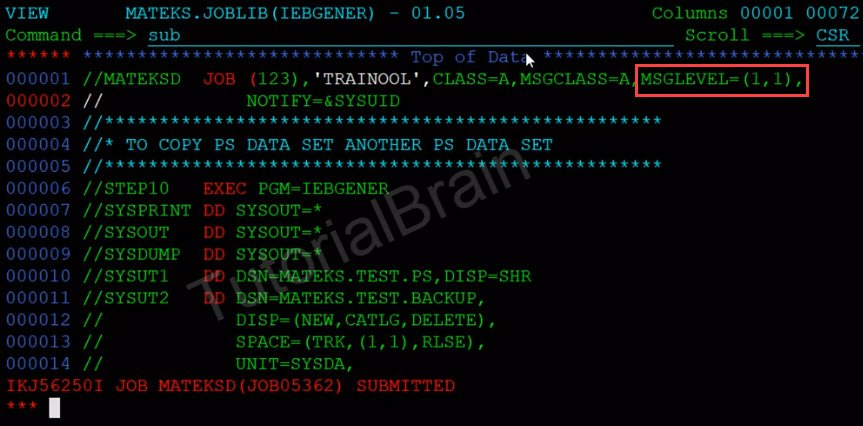# JCL MSGLEVEL Parameter

## JCL MSGLEVEL Parameter

MSGLEVEL is an optional Keyword parameter so if you do not define this parameter, then the MVS operating system will by define this MSGLEVEL by default.

Use MSGLEVEL to inform the JCL about the messages that we need to print and to specify the device in the MSGCLASS parameter.

The Syntax for MSGLEVEL:
MSGLEVEL=(JESJCL,JESYSMSG)

Here, JESJCL can take the value of 0, 1 and 2 and message can take a value of 0 or 1.

So, Let us understand what value you should code in your JESJCL

• Use value ‘0’ to print job-related statements.
• If we have code the value of 1 in JESJCL, then it prints all the JCL statements along with the procedures. If there are no procedures in a JCL, then it will only print all the JCL statements.
• The next value we have in JESJCLis 2. So, 2 prints only input JCL statement

So, Let us understand what value you should code in your JESYSMSG

• 0 means it will not show any messages except the return code. So, if the allocation or termination happens then all the allocation and termination related messages will print if the job terminates abnormally. So, if there is an ABEND (which is abnormal termination of a job) and if you have given the value of 0 then the message related to the termination will be printed in the spool.
• If you give a value of 1. So, 1 means it will show all the messages. Either, if it is an allocation or termination, irrespective of that, all the details will be displayed in the spool.

## Example when we give MSGLEVEL=(1,1)

//MATEKSD  JOB  (123),’TRAINOOL’,CLASS=A,MSGCLASS=A,MSGLEVEL=(1,1)## OutputIf you see the output in JESJCL, you will see that lot of details about the JCL is present when you give option 1 for JESJCL as below-If you see the output in JESSYSMSG, you will see that lot of system related messages is present when you give option 1 for JESYSMSG as below-## Example when we give MSGLEVEL=(1,0)

//MATEKSD  JOB  (123),’TRAINOOL’,CLASS=A,MSGCLASS=A,MSGLEVEL=(1,0)## Output

If you see the output in JESSYSMSG, you will see that few system related messages are present when you give option o for JESYSMSG. This is because if you code option 0 for SYSYSMSG and there is no abnormal termination, then few system related messages will be shown as below-## Possible combination in MSGLEVEL Parameter

There are 6 possible combination for MSGLEVEL Parameter-

1. MSGLEVEL=(0,0)
2. MSGLEVEL=(0,1)
3. MSGLEVEL=(1,0)
4. MSGLEVEL=(1,1)
5. MSGLEVEL=(2,0)
6. MSGLEVEL=(2,1)